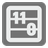Binary Count
Elementary+
ES FR HU IT JA English RU UK
We have prepared a set of Editor's Choice Solutions. You will see them first after you solve the mission. In order to see all other solutions you should change the filter.For the Robots the decimal format is inconvenient. If they need to count to "1", their computer brains want to count it in the binary representation of that number. You can read more about binary here.

You are given a number (a positive integer). You should convert it to the binary format and count how many unities (1) are in the number spelling. For example: 5 = 0b101 contains two unities, so the answer is 2.

Input: A number as a positive integer.

Output: The quantity of unities in the binary form as an integer.

Example:

```checkio(4) == 1
checkio(15) == 4
checkio(1) == 1
checkio(1022) == 9
```

How it is used: How to convert a number to the binary form. It can be useful for Computer Science purposes.

Precondition: 0 < number ≤ 232

Guido van Rossum, the author of Python, is one of our most famous player. He is writing some really wonderful code reviews for our player solutions.